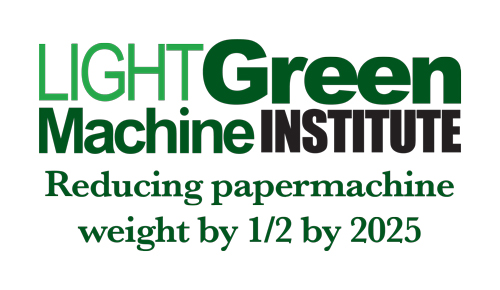The Light Green Machine InstituteNovember 2019: The Optimization Problem--Clues to Solution, Part 4By Jim Thompson   Errata... Last month I incorrectly stated that the diameter of a circle is 2 x pi when it is 2 x r (r = radius).  Fortunately, this did not affect any of the calculations, but as stated at the beginning, it was just wrong. This month, we want to introduce Dynamic Length into our machine. Dynamic Length, or DL, is related to capacity weight) and basis weight.   It is also related to the following dimensions we have already defined: W = trim width F = Fourdrinier Length PD = Pre Dryer Section Length PS = Post Size Press Dryer Section Length W is a static dimension F, PD, and PS may be dynamic dimensions, for their length depends on capacity at a given speed. As dynamic dimensions, we will express them as Fd PDd PSd We need a couple more definitions as well: Bw = Basis Weight B = Weight Ua = Unit Area Hence, Bw = B/Ua RP = Running Length of Paper/minute = Speed Then, production per minute = B/M = RP x W x B/Ua And Daily Production is 1440 x (RP x W x B/Ua) (24 hours = 1440 minutes) However, RP is constrained by 4 items: 1. Maximum mechanical design speed of machine. 2. Minimum dwell time in the Fourdrinier 3. Minimum dwell time in the Pre Dryer Section 4. Minimum dwell time in the Post Size Press Dryer Section I think that is enough to chew on for this month! As always, check my math and logic.  To be continued...